## Example 81.4 Testing a Binomial Proportion

This example tests a binomial proportion by using a four-stage group sequential design. Suppose a supermarket is developing a new store-brand coffee. From past studies, the positive response for the current store-brand coffee from customers is around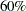. The store is interested in whether the new brand has a better positive response than the current brand.

A power family method is used for the group sequential trial with the null hypothesis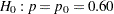and a one-sided upper alternative with a power of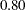at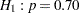. To accommodate the zero null reference that is assumed in the SEQDESIGN procedure, an equivalent hypothesiswith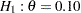is used, where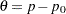. The following statements request a power family method with early stopping to reject the null hypothesis:

```ods graphics on;
proc seqdesign altref=0.10
boundaryscale=mle
;
PowerFamily: design method=pow
nstages=4
alt=upper
beta=0.20
;
samplesize model=onesamplefreq( nullprop=0.6);
ods output Boundary=Bnd_Prop;
run;
ods graphics off;
```

The NULLPROP= option in the SAMPLESIZE statement specifies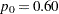for the sample size computation. The ODS OUTPUT statement with the BOUNDARY=BND_PROP option creates an output data set named BND_PROP which contains the resulting boundary information for the subsequent sequential tests.

With the BOUNDARYSCALE=MLE option, the procedure displays the output boundaries in terms of the maximum likelihood estimates. The "Design Information" table in Output 81.4.1 displays design specifications and derived statistics. With the specified alternative reference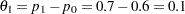, the maximum information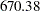is also derived.

The SEQDESIGN Procedure
Design: PowerFamily

Statistic Distribution Normal
Boundary Scale MLE
Alternative Hypothesis Upper
Early Stop Reject Null
Method Power Family
Boundary Key Both
Alternative Reference 0.1
Number of Stages 4
Alpha 0.05
Beta 0.2
Power 0.8
Max Information (Percent of Fixed Sample) 108.4306
Max Information 670.3782
Null Ref ASN (Percent of Fixed Sample) 106.9276
Alt Ref ASN (Percent of Fixed Sample) 78.51072

The "Boundary Information" table in Output 81.4.2 displays the information level, alternative reference, and boundary values at each stage. With the STOP=REJECT option, only the rejection boundary values are displayed.

Output 81.4.2 Boundary Information
Boundary Information (MLE Scale)
Null Reference = 0
_Stage_   Alternative Boundary Values
Information Level Reference Upper
Proportion Actual N Upper Alpha
1 0.2500 167.5945 35.19485 0.10000 0.20018
2 0.5000 335.1891 70.38971 0.10000 0.11903
3 0.7500 502.7836 105.5846 0.10000 0.08782
4 1.0000 670.3782 140.7794 0.10000 0.07077

With ODS Graphics enabled, a detailed boundary plot with the rejection and acceptance regions is displayed, as shown in Output 81.4.3.

Output 81.4.3 Boundary PlotWith the MODEL=ONESAMPLEFREQ option in the SAMPLESIZE statement, the "Sample Size Summary" table in Output 81.4.4 displays the parameters for the sample size computation.

Output 81.4.4 Required Sample Size Summary
Sample Size Summary
Test One-Sample Proportion
Null Proportion 0.6
Proportion 0.7
Test Statistic Z for Proportion
Reference Proportion Alt Ref
Max Sample Size 140.7794
Expected Sample Size (Null Ref) 138.828
Expected Sample Size (Alt Ref) 101.9333

The "Sample Sizes" table in Output 81.4.5 displays the required sample sizes for the group sequential clinical trial.

Output 81.4.5 Required Sample Sizes
Sample Sizes (N)
One-Sample Z Test for Proportion
_Stage_ Fractional N Ceiling N
N Information N Information
1 35.19 167.6 36 171.4
2 70.39 335.2 71 338.1
3 105.58 502.8 106 504.8
4 140.78 670.4 141 671.4

Thus,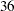customers are needed at stage, and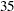new customers are needed at each of the remaining stages. Suppose thatcustomers are available at stage. Output 81.4.6 lists the 10 observations in the data set count_1.

Output 81.4.6 Clinical Trial Data
 First 10 Obs in the Trial Data

Obs Resp
1 1
2 1
3 0
4 0
5 1
6 1
7 0
8 1
9 1
10 1

The Resp variable is an indicator variable with a value offor a customer with a positive response and a value offor a customer without a positive response.

The following statements use the MEANS procedure to compute the mean response at stage:

```proc means data=Prop_1;
var Resp;
ods output Summary=Data_Prop1;
run;
```

The following statements create and display (in Output 81.4.7) the data set for the centered mean positive response,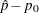:

```data Data_Prop1;
set Data_Prop1;
_Scale_='MLE';
_Stage_= 1;
NObs= Resp_N;
PDiff= Resp_Mean - 0.6;
keep _Scale_ _Stage_ NObs PDiff;
run;
proc print data=Data_Prop1;
title 'Statistics Computed at Stage 1';
run;
```

Output 81.4.7 Statistics Computed at Stage 1
 Statistics Computed at Stage 1

Obs _Scale_ _Stage_ NObs PDiff
1 MLE 1 36 -0.016667

The following statements invoke the SEQTEST procedure to test for early stopping at stage:

```ods graphics on;
proc seqtest Boundary=Bnd_Prop
Data(Testvar=PDiff)=Data_Prop1
boundarykey=both
boundaryscale=mle
;
ods output Test=Test_Prop1;
run;
ods graphics off;
```

The BOUNDARY= option specifies the input data set that provides the boundary information for the trial at stage, which was generated in the SEQDESIGN procedure. The DATA=DATA_PROP1 option specifies the input data set DATA_PROP1 that contains the test statistic and its associated sample size at stage, and the TESTVAR=PDIFF option identifies the test variable PDIFF in the data set.

If the computed information level for stageis not the same as the value provided in the BOUNDARY= data set, the INFOADJ=PROP option (which is the default) proportionally adjusts the information levels at future interim stages from the levels provided in the BOUNDARY= data set. The BOUNDARYKEY=BOTH option maintains both theandlevels. The BOUNDARYSCALE=MLE option displays the output boundaries in terms of the MLE scale.

The ODS OUTPUT statement with the TEST=TEST_PROP1 option creates an output data set named TEST_PROP1 which contains the updated boundary information for the test at stage. The data set also provides the boundary information that is needed for the group sequential test at the next stage.

The "Design Information" table in Output 81.4.8 displays design specifications. With the specified BOUNDARYKEY=BOTH option, the information levels and boundary values at future stages are modified to maintain both theandlevels.

The SEQTEST Procedure

BOUNDARY Data Set WORK.BND_PROP
Data Set WORK.DATA_PROP1
Statistic Distribution Normal
Boundary Scale MLE
Alternative Hypothesis Upper
Early Stop Reject Null
Number of Stages 4
Alpha 0.05
Beta 0.2
Power 0.8
Max Information (Percent of Fixed Sample) 108.4795
Max Information 670.680662
Null Ref ASN (Percent of Fixed Sample) 106.9693
Alt Ref ASN (Percent of Fixed Sample) 78.44835

The "Test Information" table in Output 81.4.9 displays the boundary values for the test statistic with the specified MLE scale.

Output 81.4.9 Sequential Tests
Test Information (MLE Scale)
Null Reference = 0
_Stage_   Alternative Boundary Values Test
Information Level Reference Upper PDiff
Proportion Actual N Upper Alpha Estimate Action
1 0.2556 171.4286 35.98376 0.10000 0.19638 -0.01667 Continue
2 0.5037 337.8459 70.91565 0.10000 0.11843 .
3 0.7519 504.2633 105.8475 0.10000 0.08770 .
4 1.0000 670.6807 140.7794 0.10000 0.07080 .

The information level at stageis computed as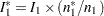, where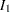and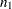are the information level and sample size at stagein the BOUNDARY= data set, and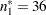is the available sample size at stage.

With the INFOADJ=PROP option (which is the default), the information levels at interim stagesandare derived proportionally from the information levels in the BOUNDARY= data set. At stage, the statistic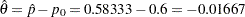is less than the upperboundary value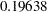, so the trial continues to the next stage.

With ODS Graphics enabled, a boundary plot with the rejection and acceptance regions is displayed, as shown in Output 81.4.10. As expected, the test statistic is in the continuation region.

Output 81.4.10 Sequential Test Plot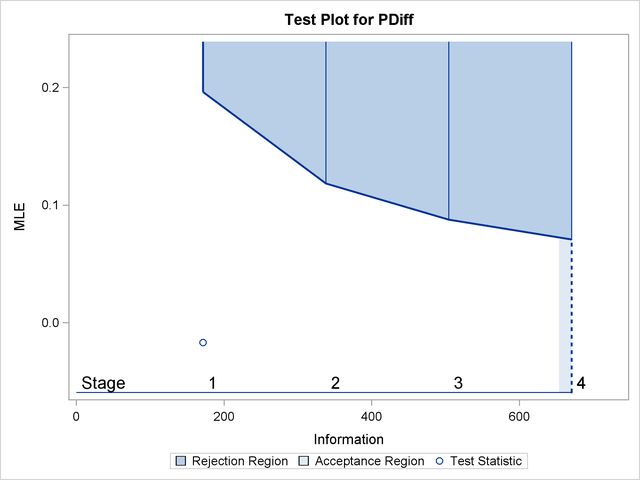The following statements use the MEANS procedure to compute the mean response at stage:

```proc means data=Prop_2;
var Resp;
ods output Summary=Data_Prop2;
run;
```

The following statements create and display (in Output 81.4.11) the data set for the centered mean positive response () at stage:

```data Data_Prop2;
set Data_Prop2;
_Scale_='MLE';
_Stage_= 2;
NObs= Resp_N;
PDiff= Resp_Mean - 0.6;
keep _Scale_ _Stage_ NObs PDiff;
run;

proc print data=Data_Prop2;
title 'Statistics Computed at Stage 2';
run;
```

Output 81.4.11 Statistics Computed at Stage 2
 Statistics Computed at Stage 2

Obs _Scale_ _Stage_ NObs PDiff
1 MLE 2 71 -0.064789

The following statements invoke the SEQTEST procedure to test for early stopping at stage:

```ods graphics on;
proc seqtest Boundary=Test_Prop1
Data(Testvar=PDiff)=Data_Prop2
boundarykey=both
boundaryscale=mle
condpower(cref=1)
predpower
plots=condpower
;
ods output test=Test_Prop2;
run;
ods graphics off;
```

The BOUNDARY= option specifies the input data set that provides the boundary information for the trial at stage, which was generated by the SEQTEST procedure at the previous stage. The DATA= option specifies the input data set that contains the test statistic and its associated sample size at stage, and the TESTVAR= option identifies the test variable in the data set.

The ODS OUTPUT statement with the TEST=TEST_PROP2 option creates an output data set named TEST_PROP2 which contains the updated boundary information for the test at stage. The data set also provides the boundary information that is needed for the group sequential test at the next stage.

The CONDPOWER(CREF=1) option requests the conditional power with the observed statistic under the alternative hypothesis, in addition to the conditional power under the hypothetical reference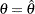, the MLE estimate. The PREDPOWER option requests the noninformative predictive power with the observed statistic.

The "Test Information" table in Output 81.4.12 displays the boundary values for the test statistic with the specified MLE scale. The test statistic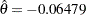is less than the corresponding upperboundary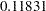, so the sequential test does not stop at stageto reject the null hypothesis.

Output 81.4.12 Sequential Tests
The SEQTEST Procedure

Test Information (MLE Scale)
Null Reference = 0
_Stage_   Alternative Boundary Values Test
Information Level Reference Upper PDiff
Proportion Actual N Upper Alpha Estimate Action
1 0.2556 171.4286 35.98223 0.10000 0.19638 -0.01667 Continue
2 0.5043 338.2478 70.99698 0.10000 0.11831 -0.06479 Continue
3 0.7522 504.4785 105.8882 0.10000 0.08767 .
4 1.0000 670.7092 140.7794 0.10000 0.07081 .

With ODS Graphics enabled, the "Test Plot" displays boundary values of the design and the test statistic, as shown in Output 81.4.13. It also shows that the test statistic is in the "Continuation Region" below the upperboundary value at stage.

Output 81.4.13 Sequential Test Plot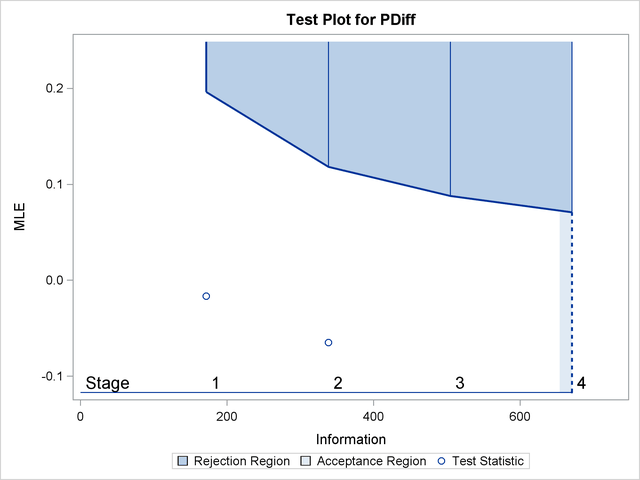The predictive power is the probability to reject the null hypothesis under the posterior distribution with a noninformative prior given the observed statistic. The "Predictive Power Information" table in Output 81.4.14 indicates that the predictive power atis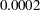.

Output 81.4.14 Predictive Power
Predictive Power Information
Stopping
Stage
MLE Predictive
Power
2 -0.06479 0.00020

The "Conditional Power Information" table in Output 81.4.15 displays conditional powers given the observed statistic under hypothetical references, the maximum likelihood estimate, and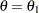. The constantunder CRef for the MLE is derived from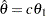; that is,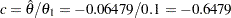.

Output 81.4.15 Conditional Power
Conditional Power Information
Reference = CRef * (Alt Reference)
Stopping
Stage
MLE Reference Conditional
Power
Ref CRef
2 -0.06479 MLE -0.6479 0.00000
2 -0.06479 Alternative 1.0000 0.02368

The conditional power is the probability of rejecting the null hypothesis under these hypothetical references given the observed statistic. The table in Output 81.4.15 shows a weak conditional power of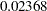under the alternative hypothesis.

The "Conditional Power Plot" displays conditional powers given the observed statistic under various hypothetical references, as shown in Output 81.4.16. These references include, the maximum likelihood estimate, and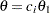, where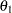is the alternative reference and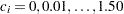are constants that are specified in the CREF= option. Output 81.4.16 shows that the conditional power increases as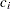increases.

Output 81.4.16 Conditional Power Plot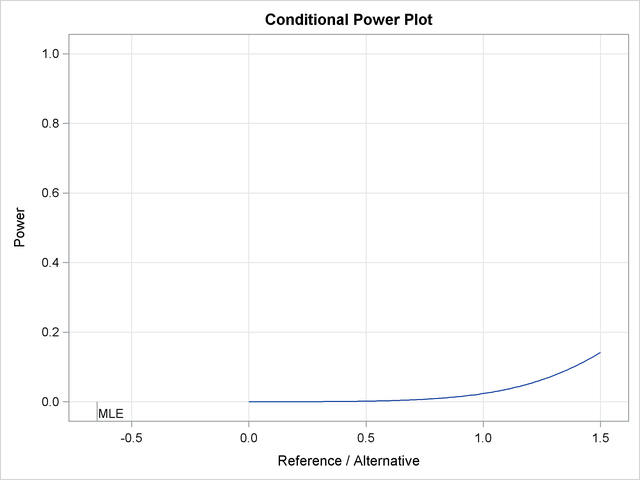With a predictive powerand a conditional power ofunder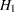, the supermarket decides to stop the trial and accept the null hypothesis. That is, the positive response for the new store-brand coffee is not better than that for the current store-brand coffee.

Output 81.4.17 Predictive Power
Predictive Power Information
Stopping
Stage
MLE Predictive
Power
2 -0.06479 0.00020

In the SEQTEST procedure, the conditional probability at an interim stageis the probability that the test statistic at the final stage (stage) would exceed the rejection critical value. Since an interim stage exists between the current stage (stage) and the final stage, the conditional power is not the conditional probability to reject the null hypothesis.

The following statements invoke the SEQTEST procedure to test for early stopping at stage. The NSTAGES=3 option sets the next stage as the final stage (stage), and the BOUNDARYKEY=BOTH option derives the information level at stagethat maintain both Type I and Type II error probability levels. The CONDPOWER(CREF=1) option requests the conditional power with the observed statistic under the alternative hypothesis, in addition to the conditional power under the hypothetical reference, the MLE estimate.

```proc seqtest Boundary=Test_Prop1
Data(Testvar=PDiff)=Data_Prop2
nstages=3
boundarykey=both
boundaryscale=mle
condpower(cref=1)
;
run;
```

The "Test Information" table in Output 81.4.18 displays the boundary values for the test statistic with the specified MLE scale, assuming that the next stage is the final stage.

Output 81.4.18 Sequential Tests
The SEQTEST Procedure

Test Information (MLE Scale)
Null Reference = 0
_Stage_   Alternative Boundary Values Test
Information Level Reference Upper PDiff
Proportion Actual N Upper Alpha Estimate Action
1 0.2645 171.4286 37.23405 0.10000 0.19638 -0.01667 Continue
2 0.5219 338.2478 73.46696 0.10000 0.11831 -0.06479 Continue
3 1.0000 648.1598 140.7794 0.10000 0.06831 .

The "Conditional Power Information" table in Output 81.4.19 displays conditional powers given the observed statistic, assuming that the next stage is the final stage.

Output 81.4.19 Conditional Power
Conditional Power Information
Reference = CRef * (Alt Reference)
Stopping
Stage
MLE Reference Conditional
Power
Ref CRef
2 -0.06479 MLE -0.6479 0.00000
2 -0.06479 Alternative 1.0000 0.02278

The conditional power is the probability of rejecting the null hypothesis under these hypothetical references given the observed statistic. The table in Output 81.4.19 also shows a weak conditional power of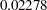under the alternative hypothesis.